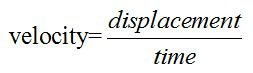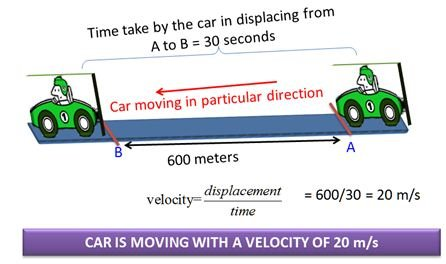# Definition Of Velocity

## Definition Of Velocity

The displacement of an object in a specific time is known as velocity
(Or) Speed of an object in particular direction.#### Example:• Displacement is measured in meters and time in seconds hence velocity is measured in m/s.
• Displacement is the path covered by a body in a particular direction. Therefore velocity is the speed of a body in particular direction.
• Direction of the body is must for measuring the velocity.
• Velocity of an object is high when an object covers large displacement in short interval of time.

#### Worked Example:

An object displaces 300 meters in 30 seconds. What is the velocity of the object?

A. 100 m/s
B. 10 m/s
C. 30 m/s
D. 1 m/s